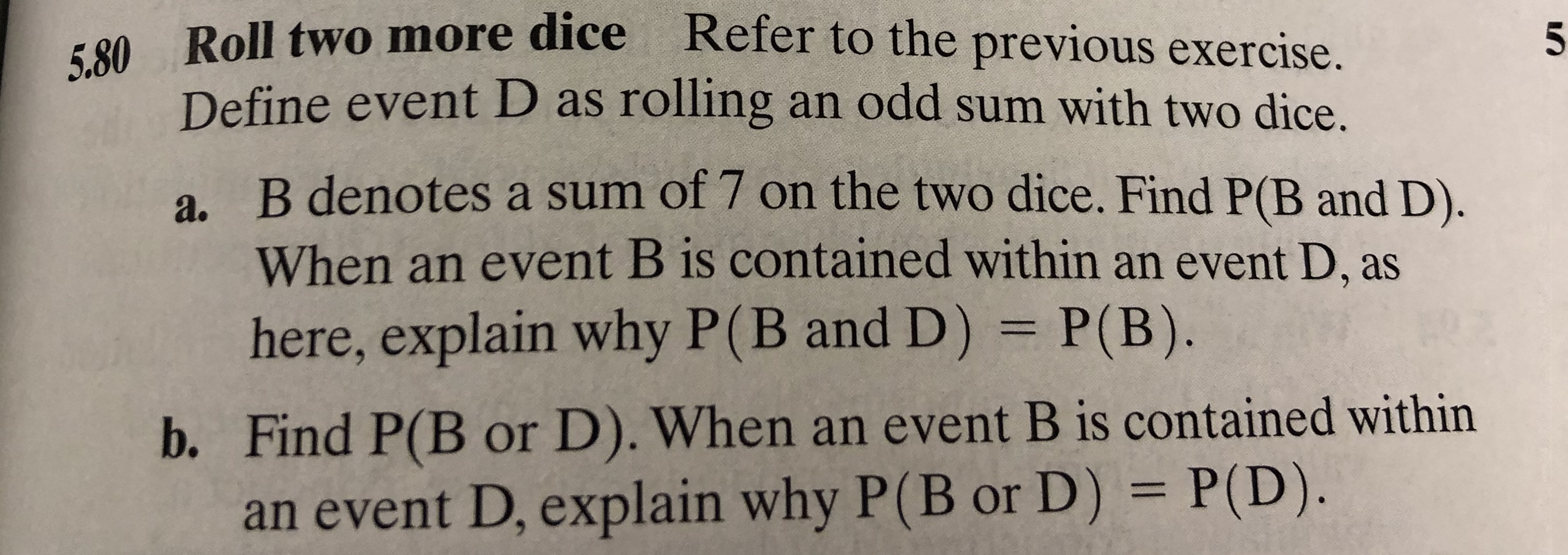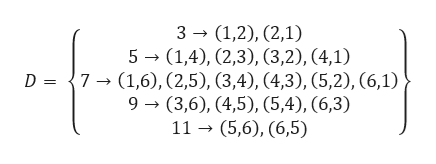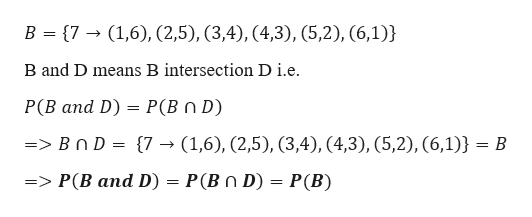# Roll two more diceRefer to the previous exercise.5.805Define event D as rolling an odd sum with two dice.a. B denotes a sum of 7 on the two dice. Find P(B and D).When an event B is contained within an event D, ashere, explain why P(B and D) P(B).b. Find P(B or D). When an event B is contained withinan event D, explain why P(B or D) = P(D).

Question
140 views

Refering to 5.79help_outlineImage TranscriptioncloseRoll two more dice Refer to the previous exercise. 5.80 5 Define event D as rolling an odd sum with two dice. a. B denotes a sum of 7 on the two dice. Find P(B and D). When an event B is contained within an event D, as here, explain why P(B and D) P(B). b. Find P(B or D). When an event B is contained within an event D, explain why P(B or D) = P(D). fullscreen
check_circle

Step 1

As per the question

Event D-> rolling an odd sum with two dice

Events in D include sum of 3,5,7,9,11help_outlineImage Transcriptionclose3(1,2), (2,1) 5 (1,4), (2,3), (3,2), (4,1) D =7(1,6), (2,5), (3,4), (4,3), (5,2), (6,1) 9 (3,6), (4,5), (5,4), (6,3) 11 (5,6), (6,5) fullscreen
Step 2

a)

Event B denotes a sum of 7 on the two...help_outlineImage TranscriptioncloseB (7(1,6), (2,5), (3,4), (4,3), (5,2), (6,1)} B and D means B intersection D i.e Р(В апd D) — Р (Вn D) => BnD= {7- (1,6), (2,5), (3,4), (4,3), (5,2), (6,1)} В => P(B and D) P(Bn D) = P(B) fullscreen

### Want to see the full answer?

See Solution

#### Want to see this answer and more?

Solutions are written by subject experts who are available 24/7. Questions are typically answered within 1 hour.*

See Solution
*Response times may vary by subject and question.
Tagged in

### Measures